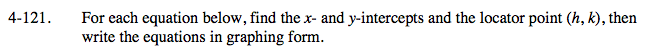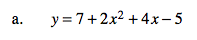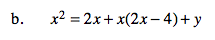### Home > A2C > Chapter 4 > Lesson 4.2.4 > Problem4-121

4-121.y-intercept: y = 7 + 2(0)2 + 4(0) − 5

You will need to factor. There is only one x-intercept.

x-intercept: 0 = 7 + 2x2 + 4x − 5

Since there is only one x-intercept, the vertex must be at the x-intercept. To find the equation in graphing form, use the equation y = a(xh)2 +k.

y-intercept: (0, 2)
x-intercept: (−1, 0)
vertex: (−1, 0)
y = 2(x + 1)2Start by finding the x- and y-intercepts.

To find the x-coordinate of the vertex, average the x-intercepts.

To find the y-coordinate of the vertex, substitute the x-coordinate of the vertex into the given equation and solve for y.

Now write the equation in graphing form.